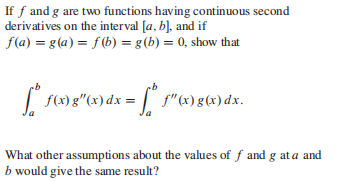### Still have math questions?If $$f$$ and $$g$$ are two functions having continuous second derivatives on the interval $$[ a , b ]$$ , and if $$f ( a ) = g ( a ) = f ( b ) = g ( b ) = 0$$ , show that $$\int _ { a } ^ { b } f ( x ) g ^ { \prime \prime } ( x ) d x = \int _ { a } ^ { b } f ^ { \prime \prime } ( x ) g ( x ) d x$$
$$\left. \begin{array} { l } { \text { Any conditions which guarantee that } } \\ { f ( b ) g ^ { \prime } ( b ) - f ^ { \prime } ( b ) g ( b ) = f ( a ) g ^ { \prime } ( a ) - f ^ { \prime } ( a ) g ( a ) } \\ { \text { will suffice. } } \end{array} \right.$$